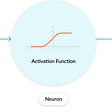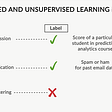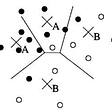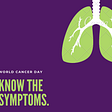# Happy Chinese New Year of the Ox 2021 !!

In this story, Reducing the Dimensionality of Data with Neural Networks, autoencoder, by University of Toronto, is briefly reviewed. This is a paper by Prof. Hinton. In this paper:

• An autoencoder is trained to reduce the data dimensions for data visualization.

This is a paper in 2006 JSCIENCE with over 14000 citations. (Sik-Ho Tsang @ Medium)

# Outline

1. Pretraining by Training Restricted Boltzmann Machine (RBM)
2. Unrolling to Form an Autoencoder
3. Fine-tuning the Autoencoder
4. Experimental Results

# 1. Pretraining by Training Restricted Boltzmann Machine (RBM)

• At the year of 2006, it was difficult to train an autoencoder with few hidden layers. A pretraining procedure is introduced by training Restricted Boltzmann Machine (RBM).
• Pretraining consists of learning a stack of restricted Boltzmann machines (RBMs), each having only one layer of feature detectors.
• The graph of an RBM has only connections between the layer of hidden and visible variables but not between two variables of the same layer.

For image, the pixels correspond to “visible” units of the RBM because their states are observed.

The feature detectors correspond to “hidden” units.

The learned feature activations of one RBM are used as the ‘‘data’’ for training the next RBM in the stack.

• A joint configuration (v, h) of the visible and hidden units has an energy:
• where vi and hj are the binary states of pixel i and feature j, bi and bj are their biases, and wij is the weight between them.
• The network assigns a probability to every possible image via this energy function.
• The RBM can be interpreted as a stochastic neural network, where nodes and edges correspond to neurons and synaptic connections, respectively.
• The conditional probability of a single variable being one can be interpreted as the firing rate of a (stochastic) neuron with sigmoid activation function 1/(1+e^(-x)):
• (The details of RBM is not covered as it can be going into very details.)

To be brief, training a whole autoencoder was difficult in the year of 2006 since there are too many layers. Pretraining RBMs can help to train the autoencoder layer by layer.

# 2. Unrolling to Form an Autoencoder

• After the pretraining, the RBMs are ‘‘unrolled’’ to create a deep autoencoder, which is then fine-tuned using backpropagation of error derivatives.

# 3. Fine-tuning the Autoencoder

• The global fine-tuning stage is then performed using backpropagation through the whole autoencoder to fine-tune the weights.
• The fine-tuning stage of the learning is to minimize the cross-entropy error:

# 4. Experimental Results

## 4.1. Image Reconstruction

• A (Top-to-bottom): Random samples of curves from the test data set; reconstructions produced by the (28×28)-400–200–100–50–25–6 6-dimensional deep autoencoder; reconstructions by “logistic PCA” using 6 components; reconstructions by logistic PCA and standard PCA using 18 components.
• The average squared error per image for the last four rows is 1.44, 7.64, 2.45, 5.90 respectively.
• The autoencoder consisted of an encoder with layers of size (28×28)-400–200–100–50–25–6 and a symmetric decoder.
• The six units in the code layer were linear and all the other units were logistic. The network was trained on 20,000 images and tested on 10,000 new images.

In the figure, the reconstructed strokes by autoencoder is much clear. Also, the error is 1.44 which is much smaller than the others.

• B (Top-to-bottom): A random test image from each class; reconstructions by the 30-dimensional 784–1000–500–250–30 autoencoder; reconstructions by 30-dimensional logistic PCA and standard PCA.
• The 784–1000–500–250–30 autoencoder is trained on 60,000 images, and tested on 10,000 new images.
• The average squared errors for the last three rows are 3.00, 8.01, and 13.87.

In the figure, the reconstructed digits by autoencoder is much clear. Also, the error is 3.00 which is much smaller than the others.

• C (Top-to-bottom): Random samples from the test data set; reconstructions by the 30-dimensional autoencoder; reconstructions by 30-dimensional PCA. The average squared errors are 126 and 135.

In the figure, the reconstructed faces by autoencoder is much clear. Also, the error is 126 which is much smaller than PCA.

## 4.2. MNIST

• A two-dimensional 784–1000–500–250–2 autoencoder produced a better visualization of the data than did the first two principal components.
• Layer-by-layer pretraining can also be used for classification and regression.
• The best reported error rates are 1.6% for randomly initialized backpropagation and 1.4% for support vector machines.
• After layer-by-layer pretraining in a 784–500–500–2000–10 network, 1.2% error rate is achieved. Pretraining helps the generalization.

## 4.3. Document Retrieval

• 804,414 newswire stories are used as a vector of document-specific probabilities of the 2000 commonest word stems.
• A 2000–500–250–125–2 autoencoder is trained on half of the stories.
• When the cosine of the angle between two codes was used to measure similarity, the 2000–500–250–125–10 autoencoder clearly outperformed latent semantic analysis (LSA).
• The 2000–500–250–125–2 autoencoder produced a better visualization of the data than did the LSA.

## Reference

[2016 JSCIENCE] [Autoencoder]
Reducing the Dimensionality of Data with Neural Networks

## Data Visualization

2002 [SNE] 2006 [Autoencoder] 2007 [UNI-SNE] 2008 [t-SNE]

--

--

--

## More from Sik-Ho Tsang

PhD, Researcher. I share what I've learnt and done. :) My LinkedIn: https://www.linkedin.com/in/sh-tsang/, My Paper Reading List: https://bit.ly/33TDhxG

Love podcasts or audiobooks? Learn on the go with our new app.

## Activation functions in ML## Introduction to Machine Learning## All about YOLOs — Part4 — YOLOv3, an Incremental Improvement## Find the best data preparation method and model using a pipeline## 3 Awesome Industrial Applications of Transfer Learning## Predicting Weather Temperature Change Using Machine Learning Models## Medicine through the lens of a computer## Sik-Ho Tsang

PhD, Researcher. I share what I've learnt and done. :) My LinkedIn: https://www.linkedin.com/in/sh-tsang/, My Paper Reading List: https://bit.ly/33TDhxG

## An Introduction to Convolutional Neural Network (CNN)## Review — Learning classification with Unlabeled Data## Deep Learning Model for Lung Cancer Detection## Anomaly Detection in Machine Learning